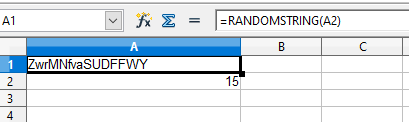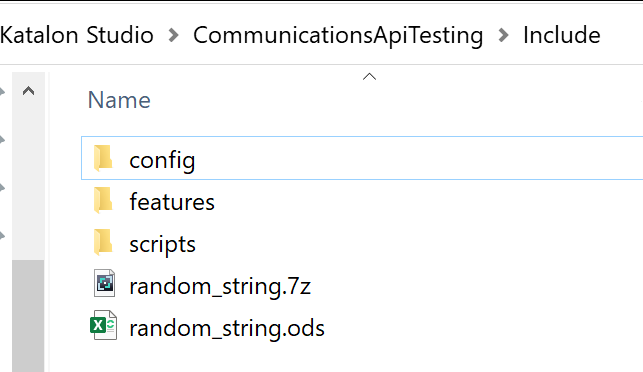# Creating a random string in Excel file to use in Katalon test case

Hi, there. I have searched the forum, bu tdidn,t find the solution for:
Creating a random string in Excel file to use in Katalon test case
I need a function to put in excel that will create anytime different string for my test case.
I prefer not to write code if it is possible :-). Thanks

I don’t think this is possible. Any data read in from an excel file will be static data. You will need to write some code to do this.

hi,

I didn’t get your point can you clarify it more
if I understood your question correct you will need a random string which is generated in excel file?
if so then need to do VBA script function which is fired when file is used

1 Like

hi,

I tried this with OO calc, looks that string is every time different when it’s opened

``````Sub Run_at_open

Dim ran As String
' ranString = RandomString(15)
' MsgBox "random string is: "+ranString
' Print "This macro runs when this file opened"

End Sub

Function RandomString(Length As Integer)
'PURPOSE: Create a Randomized String of Characters

Dim CharacterBank As Variant
Dim x As Long
Dim str As String

'Test Length Input
If Length < 1 Then
MsgBox "Length variable must be greater than 0"
Exit Function
End If

CharacterBank = Array("a", "b", "c", "d", "e", "f", "g", "h", "i", "j", _
"k", "l", "m", "n", "o", "p", "q", "r", "s", "t", "u", "v", "w", "x", _
"y", "z", "0", "1", "2", "3", "4", "5", "6", "7", "8", "9", "!", "@", _
"#", "\$", "%", "^", "&", "*", "A", "B", "C", "D", "E", "F", "G", "H", _
"I", "J", "K", "L", "M", "N", "O", "P", "Q", "R", "S", "T", "U", "V", _
"W", "X", "Y", "Z")

'Randomly Select Characters One-by-One
For x = 1 To Length
Randomize
str = str & CharacterBank(Int((UBound(CharacterBank) - LBound(CharacterBank) + 1) * Rnd + LBound(CharacterBank)))
Next x

'Output Randomly Generated String
RandomString = str

End Function
``````Uff. If you say so. So the only way to create random names is using code? Than i will take an advice with coding.

See @Timo_Kuisma1’s answer, it may work for you.

Can yuo reccomend which function will be usefull?

Thanks. Give plase the initial steps. I am not very aware of excel.

hello,

i will continue with this after my work day is over,
need to implement java code which will call the sheet and return random string

1 Like

hi,

this is hard to do without real excel app and with OO calc it’s wasted time, sorry1 Like

hi,

ok, got it to work in OpenOffice SpreadSheet application

this will generate random string between 10 to 20 chars

latest stable binary
Version 1.2 , November 9, 2010jOpenDocument-1.2-jdk5.jar (Java 5)
and add it to the your Katalon project Drivers folder
then extract this .ods file to your Katalon project Include/spreadsheet folder
random_string.7z (5.7 KB)

And here the test code

``````import java.awt.Desktop

String filename = System.getProperty("user.dir")+"\\Include\\spreadsheet\\random_string.ods";

Desktop.getDesktop().open(new File(filename));

def randString = getRandString(filename);
println randString

public static String getRandString(String filename) throws IOException {

File f = new File(filename);

//We'll assume everything we want is on the first sheet:
Sheet sheet = SpreadSheet.createFromFile(f).getSheet(0);

String cellText = "";
cellText = sheet.getCellAt(0, 0).getTextValue();

return cellText

}
``````

And in .ods file this will need to add
In .ods file
Tools → customize → Events (tab)
Open Document → Add Macro → select Run_at_openU\$ydSIX1JWdm2
9ImbhL0yoZaNu%

Thank you !

I don,t have spreadsheet folder:And please tell me where to put this code that you give meThank you!